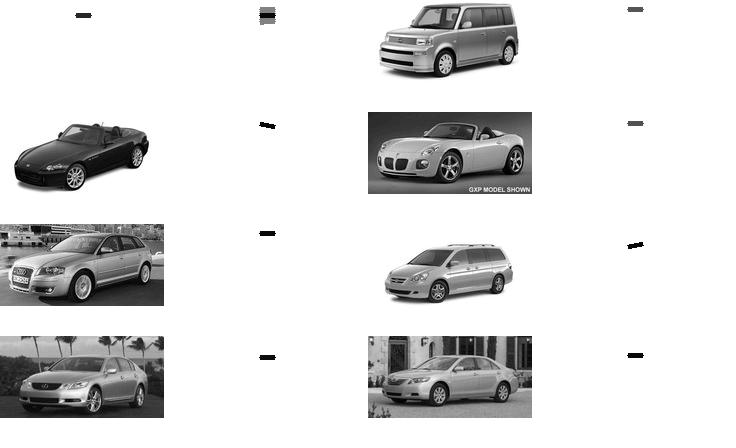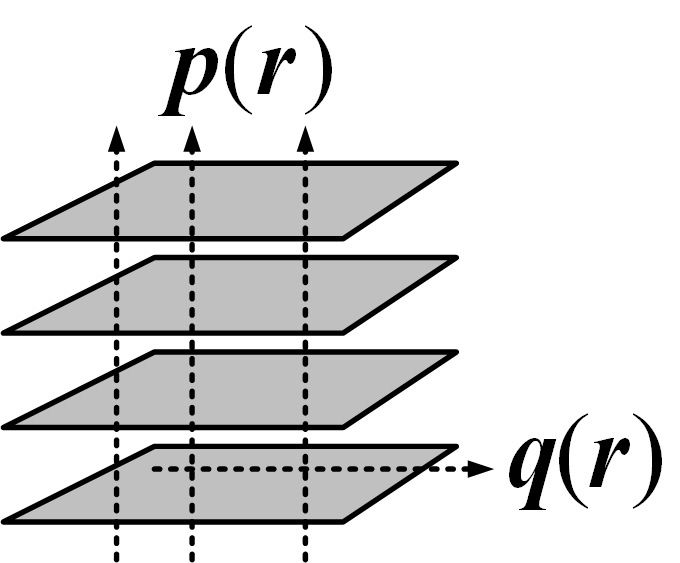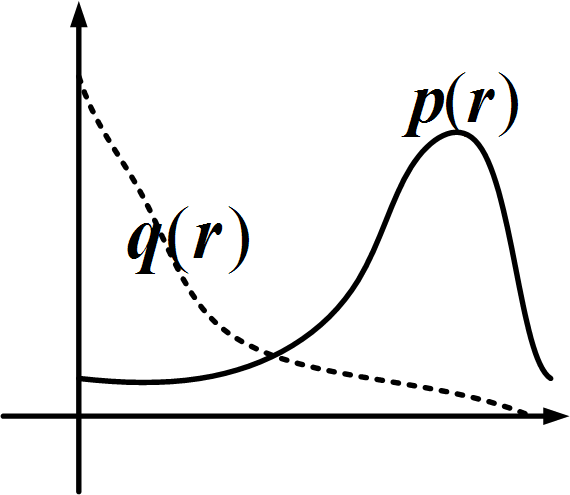# Go to reproducibility page for IJCV paper for the most updated source code and results.

The ICCV07 presentation ppt has improved experimental results and better theoretical understanding than ICCV07 paper.

Last updated on Feb 2008.

ICCV07 paper latex files
ICCV07 ppt (improved experiments and theory)
Experiments 1 and 2 (26 examples)
Experiment 3
Experiment 4
Experiment 5Active basis formed by 60 Gabor wavelet elements. The first plot displays the 60 elements, where each element is represented by a bar. For each of the other 7 pairs, the left plot is the observed image, and the right plot displays the 60 Gabor wavelet elements resulting from locally shifting the 60 elements in the first plot to fit the corresponding observed image.\$p(r_{m, 1}, ..., r_{m, n})\$ is pooled over training images (shaded rectangles) at specific locations. \$q(r_{m,1}, ..., r_{m, n})\$ is derived from stationary background \$q(\I_m)\$.

Back to active basis homepage